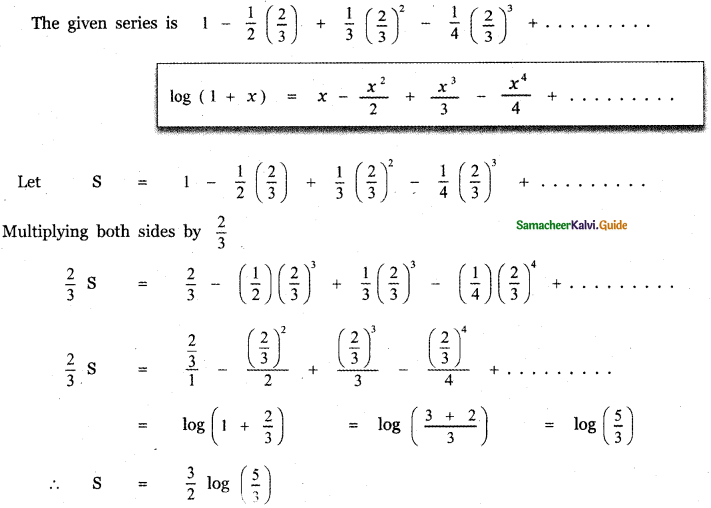Tamilnadu State Board New Syllabus Samacheer Kalvi 11th Maths Guide Pdf Chapter 5 Binomial Theorem, Sequences and Series Ex 5.5 Text Book Back Questions and Answers, Notes.

## Tamilnadu Samacheer Kalvi 11th Maths Solutions Chapter 5 Binomial Theorem, Sequences and Series Ex 5.5

Choose the correct or the most suitable answer:

Question 1.
The value of 2 + 4 + 6 + …………… + 2n is
(1) $$\frac{n(n-1)}{2}$$
(2) $$\frac{n(n+1)}{2}$$
(3) $$\frac{2 n(2 n+1)}{2}$$
(4) n(n + 1)
(4) n(n + 1)

Explaination:
2 + 4 + 6 + ……… + 2n = 2(1 + 2 + 3 + ………….. + n)
= 2 × $$\frac{n(n+1)}{2}$$
= n(n + 1)Question 2.
The coefficient of x6 in (2 + 2x)10 is
(1) 10C6
(2) 26
(3) 10C626
(4) 10C6210
(4) 10C6210

Explaination: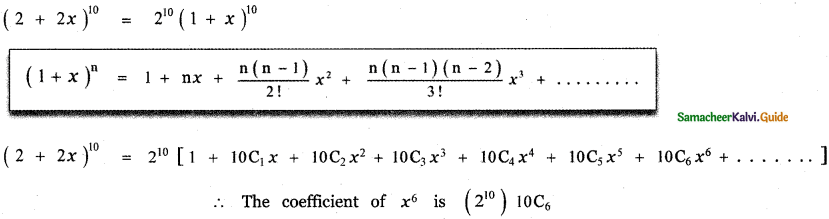Question 3.
The coefficient of x8 y12 in the expansion of (2x + 3y)20 is
(1) 0
(2) 28 312
(3) 28 312 + 212 38
(4) 20C8 28 312
(4) 20C8 28 312

Explaination:Question 4.
If nC10 > nCr for all possible r then a value of n is
(1) 10
(2) 21
(3) 19
(4) 20
(4) 20

Explaination:
Out of 10C10, 21C10, 19C10 and 20C10, 20C10 is larger.

Question 5.
If a is the Arithmetic mean and g is the Geometric mean of two numbers then
(1) a ≤ g
(2) a ≥ g
(3) a = g
(4) a > g
(2) a ≥ g

Explaination:
Given Arithmetic mean = a,
Geometric mean = g
We have A. M ≥ G. M
∴ a ≥ gQuestion 6.
If (1 + x2)2 (1 + x)n = a0 + a1x + a2x2 + ………… + xn + 4 and if a0, a1, a2 are in A. P then n is
(1) 1
(2) 2
(3) 3
(4) 4
(3) 3

Explaination:n2 – 5n + 6 = 0
(n – 2) (n – 3) = 0
n = 2 or n = 3Question 7.
If a, 8, b are in A .P , a, 4 , b are in G. P and if a, x ,b are in H . P then x is
(1) 2
(2) 1
(3) 4
(4) 16
(1) 2

Explaination:
Given a, 8, b are in A. P ∴ 2 × 8 = a + b ⇒ a + b = 16 ——— (1)
Also a, 4, b are in G.P ∴ 42 = a . b ⇒ ab = 16 ——- (2)
Also a, x, b are in H.P. ∴ $$\frac{1}{a}, \frac{1}{x}, \frac{1}{b}$$ are in A.P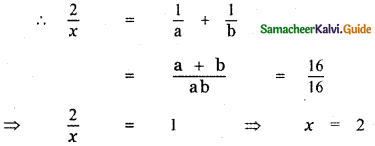Question 8.(1) A. P
(2) G.P
(3) H.P
(4) AGP
(3) H.P

Explaination:Question 9.
The H.M of two positive numbers whose A.M and G.M are 16,8 respectively is
(1) 10
(2) 6
(3) 5
(4) 4
(4) 4

Explaination:
Let a, b be the two numbers. Given A. M = $$\frac{a+b}{2}$$ = 16
G.M = $$\sqrt{a b}$$ = 8
a+b = 16 × 2 = 32
ab = 82 = 64
H.M = $$\frac{2 a b}{a+b}$$
= $$\frac{2 \times 64}{32}$$ = 2 × 2 = 4Question 10.
If S denote the sum of n terms of an A. P whose common difference is d, the value of Sn – 2Sn- 1 + Sn – 2 is
(1) d
(2) 2d
(3) 4d
(4) d2
(1) d

Explaination:= a + (n – 1) d – (a + (n – 2)d)
= a + (n – 1) d – a – (n – 2)d
= a + nd – d – a – nd + 2d = d

Question 11.
The remainder when 3815 is divided by 13 is
(1) 12
(2) 1
(3) 11
(4) 5
(1) 12

Explaination:
3815 = (39 – 1)15 = 3915 – 15C1 3914(1) + 15C2 (39)13(1)2 – 15C3 (39)12(1)3 ….. + 15C14 (39)1(1) – 15C15(1)
Except -1 all other terms are divisible by 13.
∴ When 1 is added to it the number is divisible by 13. So the remainder is 13 – 1 = 12.Question 12.
The nth term of the sequence 1, 2, 4, 7, 11, ………….. is
(1) n2 + 3n2 + 2n
(2) n3 – 3n2 + 3n
(3) $$\frac{n(n+1)(n+2)}{3}$$
(4) $$\frac{n^{2}-n+2}{2}$$
(4) $$\frac{n^{2}-n+2}{2}$$

Explaination: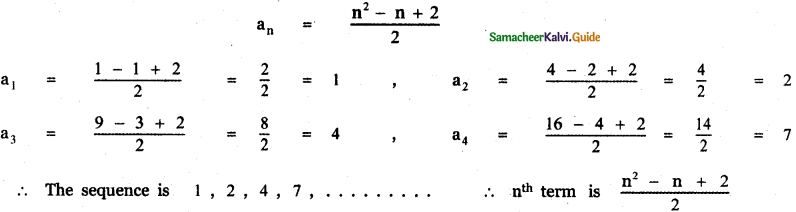Question 13.
The sum up to n terms of the series(1) $$\sqrt{2 n+1}$$
(2) $$\frac{\sqrt{2 n+1}}{2}$$
(3) $$\sqrt{2 n+1}-1$$
(4) $$\frac{\sqrt{2 n+1}-1}{2}$$
(4) $$\frac{\sqrt{2 n+1}-1}{2}$$

Explaination: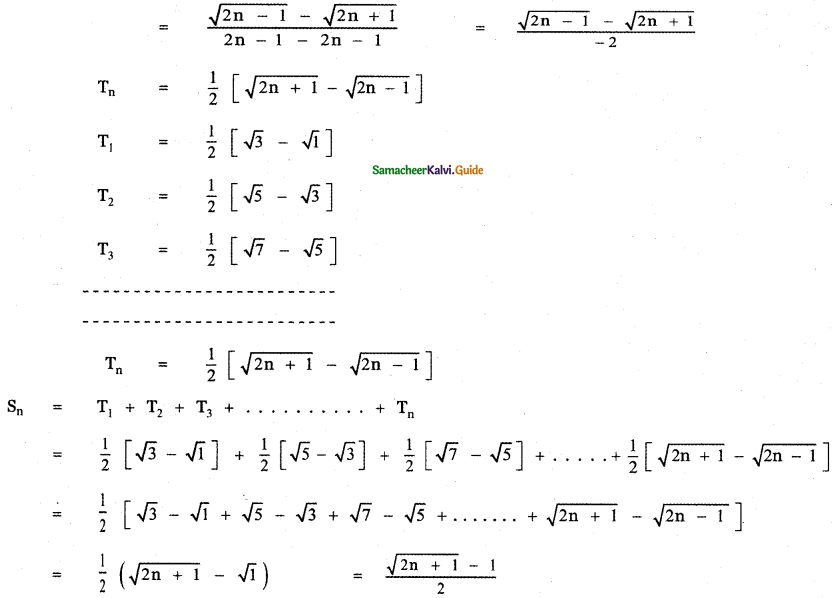Question 14.
The nth term of the sequence(1) 2n – n – 1
(2) 1 – 2-n
(3) 2-n + n – 1
(4) 2n-1
(2) 1 – 2-n

Explaination:Question 15.
The sum up to n terms of the series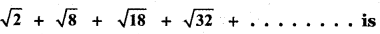(1) $$\frac{\mathbf{n}(\mathbf{n}+1)}{2}$$
(2) 2n (n + 1)
(3) $$\frac{\mathbf{n}(\mathbf{n}+1)}{\sqrt{2}}$$
(4) 1
(3) $$\frac{\mathbf{n}(\mathbf{n}+1)}{\sqrt{2}}$$

Explaination:Question 16.
The value of the series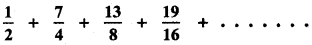(1) 14
(2) 7
(3) 4
(4) 6
(1) 14

Explaination:Question 17.
The sum of an infinite G.P is 18. If the first term is 6 the common ratio is
(1) $$\frac{1}{3}$$
(2) $$\frac{2}{3}$$
(3) $$\frac{1}{6}$$
(4) $$\frac{3}{4}$$
(2) $$\frac{2}{3}$$

Explaination:
Let the geometric series be a, ar, ar2, …………… arn-1
Given a = 6, S = 18Question 18.
The coefficient of x5 in the series e-2x is
(1) $$\frac{2}{3}$$
(2) $$\frac{3}{2}$$
(3) $$-\frac{4}{15}$$
(4) $$\frac{4}{15}$$
(3) $$-\frac{4}{15}$$

Explaination: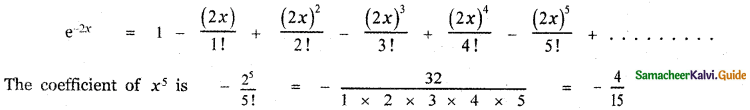Question 19.
The value of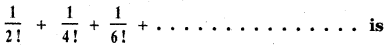(1) $$\frac{e^{2}+1}{2 e}$$
(2) $$\frac{(e+1)^{2}}{2 e}$$
(3) $$\frac{(e-1)^{2}}{2 e}$$
(4) $$\frac{e^{2}+1}{2 e}$$
(3) $$\frac{(e-1)^{2}}{2 e}$$

Explaination:Question 20.
The value of(1) log $$\left(\frac{5}{3}\right)$$
(2) $$\frac{3}{2}$$ log $$\left(\frac{5}{3}\right)$$
(3) $$\frac{5}{3}$$ log $$\left(\frac{5}{3}\right)$$
(4) $$\frac{2}{3}$$ log $$\left(\frac{2}{3}\right)$$
(2) $$\frac{3}{2}$$ log $$\left(\frac{5}{3}\right)$$